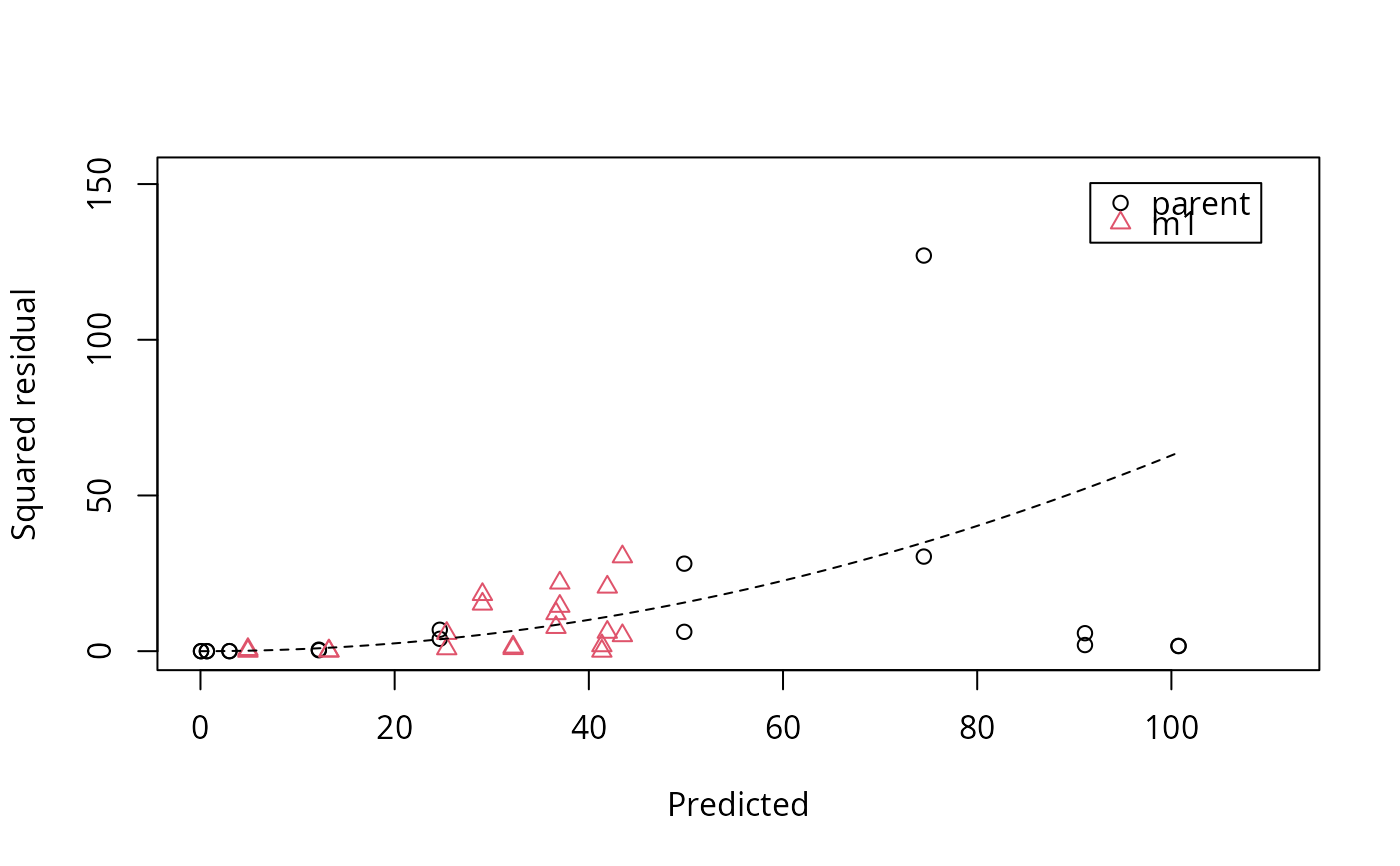This function plots the squared residuals for the specified subset of the observed variables from an mkinfit object. In addition, one or more dashed line(s) show the fitted error model. A combined plot of the fitted model and this error model plot can be obtained with plot.mkinfit using the argument show_errplot = TRUE.

mkinerrplot(
object,
obs_vars = names(object$mkinmod$map),
xlim = c(0, 1.1 * max(object$data$predicted)),
xlab = "Predicted",
ylab = "Squared residual",
maxy = "auto",
legend = TRUE,
lpos = "topright",
col_obs = "auto",
pch_obs = "auto",
frame = TRUE,
...
)

## Arguments

object

A fit represented in an mkinfit object.

obs_vars

A character vector of names of the observed variables for which residuals should be plotted. Defaults to all observed variables in the model

xlim

plot range in x direction.

xlab

Label for the x axis.

ylab

Label for the y axis.

maxy

Maximum value of the residuals. This is used for the scaling of the y axis and defaults to "auto".

legend

Should a legend be plotted?

lpos

Where should the legend be placed? Default is "topright". Will be passed on to legend.

col_obs

Colors for the observed variables.

pch_obs

Symbols to be used for the observed variables.

frame

Should a frame be drawn around the plots?

...

further arguments passed to plot.

## Value

Nothing is returned by this function, as it is called for its side effect, namely to produce a plot.

mkinplot, for a way to plot the data and the fitted lines of the mkinfit object.

Johannes Ranke

## Examples


# \dontrun{
model <- mkinmod(parent = mkinsub("SFO", "m1"), m1 = mkinsub("SFO"))
#> Temporary DLL for differentials generated and loaded
fit <- mkinfit(model, FOCUS_2006_D, error_model = "tc", quiet = TRUE)
#> Warning: Observations with value of zero were removed from the data
mkinerrplot(fit)# }